# Harnessing the Power of Math-Related Functions in Salesforce Formulas: Unlocking Advanced Calculations and Business Logic

Introduction:

Salesforce formulas provide a robust set of math-related functions that enable administrators and developers to perform complex calculations and implement intricate business logic. From basic arithmetic operations to advanced mathematical calculations, these functions empower users to automate processes and make informed decisions. In this blog post, we will explore four complex scenarios where math-related functions prove invaluable and provide step-by-step explanations on how to implement them effectively.

Understanding Important Math-Related Functions in Formulas:

Salesforce offers a comprehensive range of math-related functions that cater to various calculation needs. Here are some essential functions you need to be familiar with:

• ABS(number): Returns the absolute value of a number.
• ROUND(number, numDigits): Rounds a number to a specified number of decimal places.
• CEILING(number): Rounds a number up to the nearest integer.
• FLOOR(number): Rounds a number down to the nearest integer.
• POWER(number, exponent): Raises a number to the specified power.
• SQRT(number): Returns the square root of a number.
• EXP(number): Calculates the exponential value of a number.
• LOG(number): Returns the natural logarithm of a number.
• MAX(number1, number2, ... , numberN): Returns the highest value among a set of numbers.
• MIN(number1, number2, ... , numberN): Returns the lowest value among a set of numbers.

Now, let's explore four complex scenarios where math-related functions can be utilized effectively in Salesforce formulas:

Scenario 1: Calculating Compound Interest

In financial calculations, determining compound interest is vital for financial forecasting. Suppose you have a custom object called "Investment" with fields like "Principal_Amount__c," "Interest_Rate__c," and "Investment_Term__c." You can calculate the final investment value using the `POWER()` and `ROUND()` functions.

Implementation: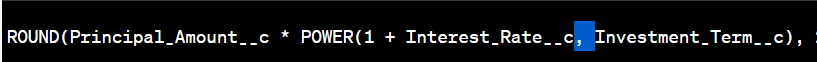ROUND(Principal_Amount__c * POWER(1 + Interest_Rate__c, Investment_Term__c), 2)

This formula raises the sum of 1 and the interest rate to the power of the investment term, multiplies it by the principal amount, and rounds the result to two decimal places.

Scenario 2: Determining Weighted Average

In data analysis, calculating weighted averages is essential for accurate statistical insights. Let's say you have a custom object called "Sales_Records" with fields like "Quantity__c" and "Unit_Price__c." You can calculate the weighted average price using the `SUM()` and `ROUND()` functions.

Implementation: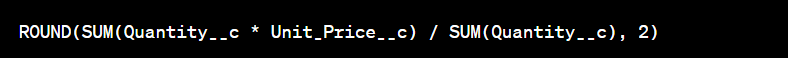ROUND(SUM(Quantity__c * Unit_Price__c) / SUM(Quantity__c), 2)

This formula multiplies the quantity and unit price for each record, sums the results, and then divides by the total quantity to determine the weighted average price.

Scenario 3: Generating Random Numbers

In certain scenarios, generating random numbers is useful for simulations, testing, or assigning random values. Salesforce provides the `RAND()` function to generate random numbers between 0 and 1.

Implementation: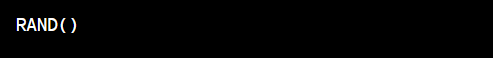RAND()

This formula generates a random number between 0 and 1 each time it is evaluated.

Scenario 4: Forecasting Revenue Growth

Forecasting revenue growth is vital for sales planning and setting targets. Let's consider a custom object called "Opportunity" with fields like "Initial_Revenue__c" and "Growth_Rate__c." You can calculate the projected revenue after a certain period using the `POWER

()` and `ROUND()` functions.

Implementation: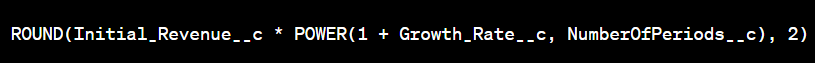ROUND(Initial_Revenue__c * POWER(1 + Growth_Rate__c, NumberOfPeriods__c), 2)

This formula raises the sum of 1 and the growth rate to the power of the number of periods, multiplies it by the initial revenue, and rounds the result to two decimal places.

Scenario 5: Calculating Sales Commission based on a Tiered Structure

In sales compensation management, calculating commissions based on a tiered structure is a common requirement. The commission percentage varies based on predefined sales thresholds. You can leverage math-related formulas to determine the commission amount dynamically based on the achieved sales target.

Implementation:

Assume you have a custom object called "Opportunity" with fields like "Amount" to capture the sales amount and "Commission__c" to store the calculated commission amount. Here's how you can implement the calculation for commission based on a tiered structure:

1. Define the Tiered Structure:

First, you need to define the commission tiers and their corresponding rates. For example, let's consider three tiers:

• - Tier 1: Sales up to \$10,000, commission rate 5%
• - Tier 2: Sales from \$10,001 to \$20,000, commission rate 7%
• - Tier 3: Sales above \$20,000, commission rate 10%

2. Calculate the Commission:

To calculate the commission amount based on the achieved sales target, you can use an IF statement along with math-related functions.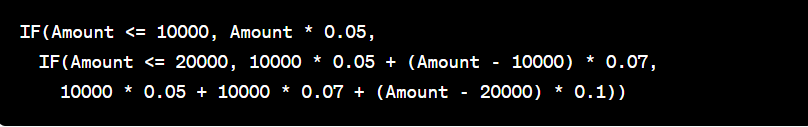``` IF(Amount <= 10000, Amount * 0.05, IF(Amount <= 20000, 10000 * 0.05 + (Amount - 10000) * 0.07, 10000 * 0.05 + 10000 * 0.07 + (Amount - 20000) * 0.1)) ```

This formula checks the sales amount (`Amount` field) against the defined tiers and calculates the commission amount accordingly. It sums the commission amounts for each tier using nested IF statements.

By using this formula, the commission amount will be dynamically calculated based on the achieved sales target and the corresponding commission rates for each tier.

Conclusion:

Math-related functions in Salesforce formulas offer a wide range of capabilities for complex calculations and business logic implementation. In this blog post, we explored four scenarios where these functions prove invaluable, ranging from calculating compound interest to forecasting revenue growth. By leveraging functions such as `POWER()`, `ROUND()`, and `SUM()`, users can automate calculations, gain valuable insights, and drive informed decision-making within their Salesforce org. Remember to consult Salesforce documentation for a comprehensive list of math-related functions and best practices to utilize their full potential. Embrace the power of math-related functions and unlock new levels of efficiency and accuracy in your Salesforce implementation.

In the final scenario discussed above, we demonstrated how to calculate the commission amount dynamically using an IF statement and math-related functions like multiplication and addition. These formulas enable organizations to automate commission calculations, incentivize sales teams effectively, and ensure accurate compensation management. Leverage the flexibility and power of math-related formulas to enhance your sales processes and drive performance in your Salesforce implementation.